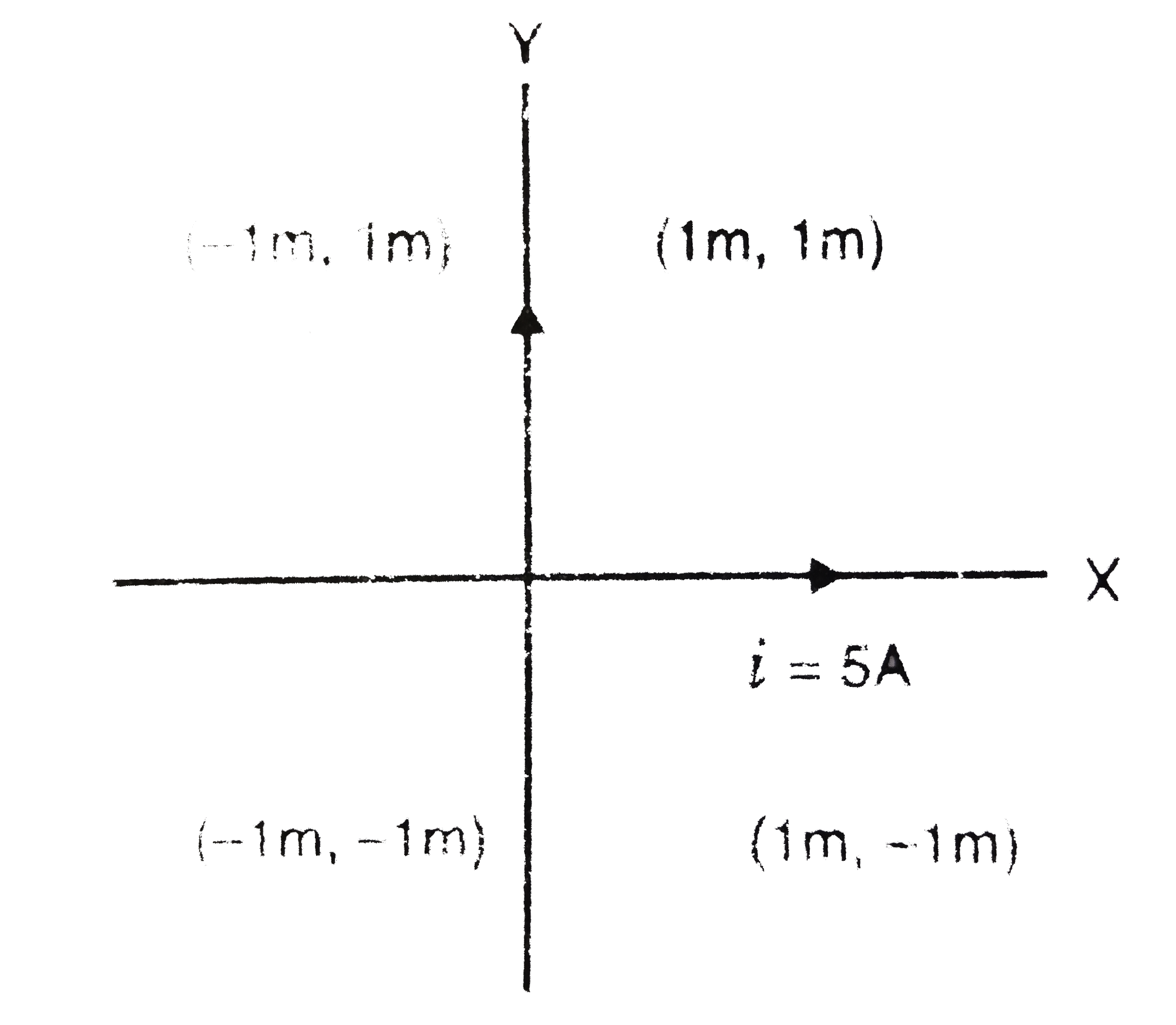Doubtnut App - Get 50 Lakh+ video solutions better than GoogleInstall Now

# Two long, straight wires, each carrying a current of 5A, are placed along the x and y axes respectively. The currents point along the positive directions of the axes. Find the magnetic fields at the points (a) (1m, 1m), (b) (-1m, 1m), (c) (-1m, -1m) and (d) (1m, -1m).

Text Solution
Verified by Experts

## (a) At point (1m,1m). Magnetic fields due to teach wire are same in magnitude but opposite in direction. Hence net magnetic field = 0 (b) At point (-1 m, 1m) magnetic field due ot these two wires =(2×10−7×51+2×10−7×51) .=2xx10^(-6)T<br>alongz−aξs=2pi T.<br>along z-axis.(c)similaras(a)≠tmag≠ticfi≤dB = 0 (d)Similaras(b),here≠tmag≠ticfi≤dare∈oppositez−directionanditsmagnitudeB = 2muT.